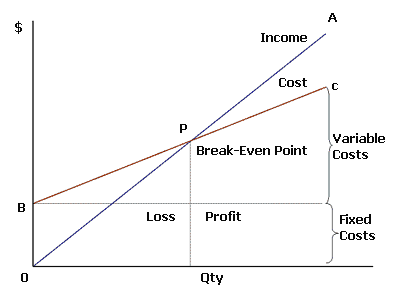You are here : > Home> Utilities > Economic and financial analysis > Break-Even Point In Quantities >

### Break-Even Point In Quantities

To determine the value of sales and quantities, at a point where there is neither profit nor loss, entrepreneurs use the technique of break-even point (BEP) or leveling point. To do this, is necessary the following elements:
a) Total fixed cost;
b) Variable cost unit;
c) Unit sale price.
Read the full text Break-even point.
Enter data as requested, using the point as a decimal separator. Ex 22,532.34 enter: 22532.34; The results will be displayed automatically after one click on Calculate.Break-Even Point Fixed cost: Variable cost per unit: Unit sale price: Break-even point in quantities: Sales volume: